## Binary Search Tree-

Before you go through this article, make sure that you have gone through the previous article on Binary Search Trees.

Binary search tree (BST) is a special kind of binary tree where each node contains-

• Only larger values in its right subtree.
• Only smaller values in its left subtree.

## Binary Search Tree Operations-

Commonly performed operations on binary search tree are-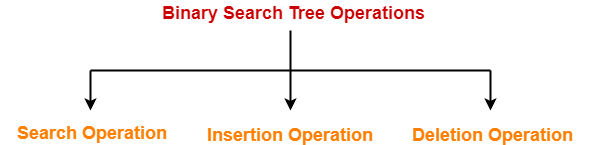1. Search Operation
2. Insertion Operation
3. Deletion Operation

## 1. Search Operation-

 Search Operation is performed to search a particular element in the Binary Search Tree.

## Rules-

For searching a given key in the BST,

• Compare the key with the value of root node.
• If the key is present at the root node, then return the root node.
• If the key is greater than the root node value, then recur for the root node’s right subtree.
• If the key is smaller than the root node value, then recur for the root node’s left subtree.

## Example-

Consider key = 45 has to be searched in the given BST-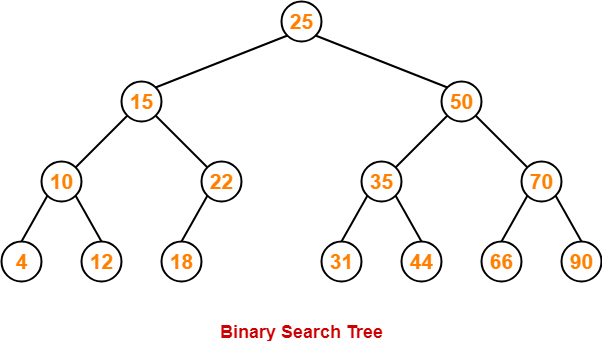• We start our search from the root node 25.
• As 45 > 25, so we search in 25’s right subtree.
• As 45 < 50, so we search in 50’s left subtree.
• As 45 > 35, so we search in 35’s right subtree.
• As 45 > 44, so we search in 44’s right subtree but 44 has no subtrees.
• So, we conclude that 45 is not present in the above BST.

## 2. Insertion Operation-

 Insertion Operation is performed to insert an element in the Binary Search Tree.

## Rules-

The insertion of a new key always takes place as the child of some leaf node.

For finding out the suitable leaf node,

• Search the key to be inserted from the root node till some leaf node is reached.
• Once a leaf node is reached, insert the key as child of that leaf node.

## Example-

Consider the following example where key = 40 is inserted in the given BST-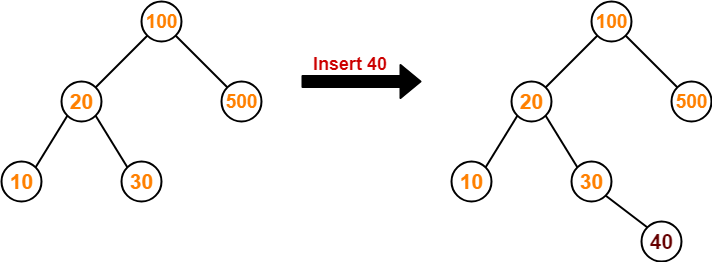• We start searching for value 40 from the root node 100.
• As 40 < 100, so we search in 100’s left subtree.
• As 40 > 20, so we search in 20’s right subtree.
• As 40 > 30, so we add 40 to 30’s right subtree.

## 3. Deletion Operation-

 Deletion Operation is performed to delete a particular element from the Binary Search Tree.

When it comes to deleting a node from the binary search tree, following three cases are possible-

### Case-01: Deletion Of A Node Having No Child (Leaf Node)-

Just remove / disconnect the leaf node that is to deleted from the tree.

### Example-

Consider the following example where node with value = 20 is deleted from the BST-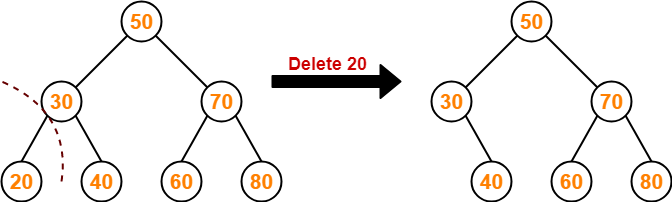### Case-02: Deletion Of A Node Having Only One Child-

Just make the child of the deleting node, the child of its grandparent.

### Example-

Consider the following example where node with value = 30 is deleted from the BST-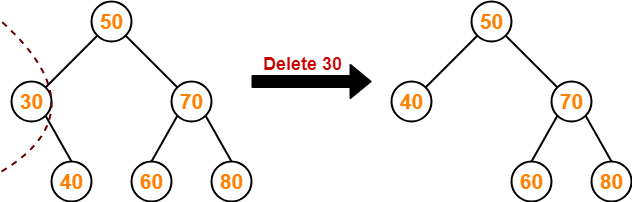### Case-02: Deletion Of A Node Having Two Children-

A node with two children may be deleted from the BST in the following two ways-

### Method-01:

• Visit to the right subtree of the deleting node.
• Pluck the least value element called as inorder successor.
• Replace the deleting element with its inorder successor.

### Example-

Consider the following example where node with value = 15 is deleted from the BST-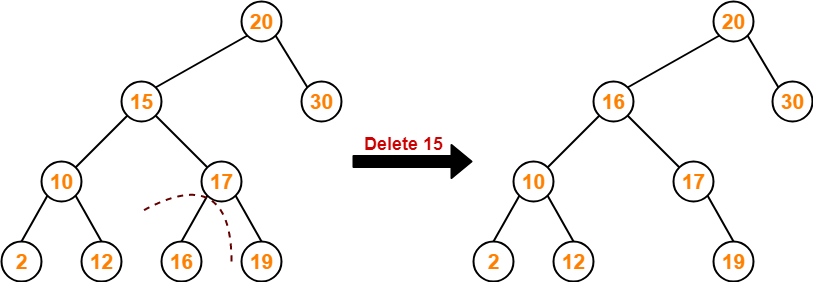### Method-02:

• Visit to the left subtree of the deleting node.
• Pluck the greatest value element called as inorder predecessor.
• Replace the deleting element with its inorder predecessor.

### Example-

Consider the following example where node with value = 15 is deleted from the BST-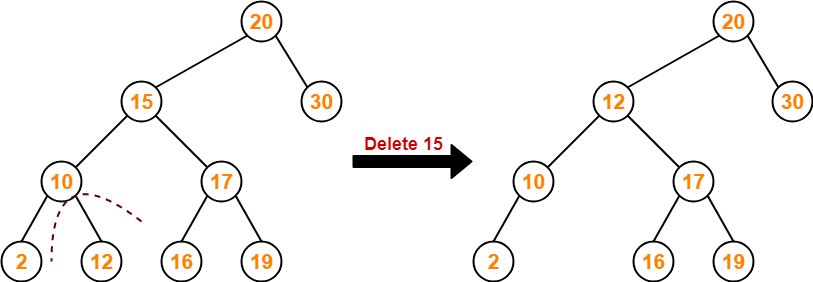To gain better understanding about BST Operations,

Watch this Video LectureNext Article- Time Complexity of BST Operations

Get more notes and other study material of Data Structures.

Watch video lectures by visiting our YouTube channel LearnVidFun.